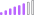cancel
Showing results for
Did you mean:

Fabric is Generally Available. Browse Fabric Presentations. Work towards your Fabric certification with the Cloud Skills Challenge.Post Patron

## What is the difference between a measure and calculated column

Hi

Simple google will show that this question has been asked many times and many videos and articls about it. However the more I read or watch video, the more I am confused. I would appreciate if you can simplfy the difference for me using simple example so I can understand the concept. Thank you very much for your help in advance.

1 ACCEPTED SOLUTIONCommunity Support

Hi, @lastnn30

Take an example.

Sample data:Calculate the overall score for each student.

Calculated column:

``````Column =
CALCULATE (
SUM ( 'Table'[Scores] ),
ALLEXCEPT('Table','Table'[Student]
))``````Measure:

``Measure = CALCULATE(SUM('Table'[Scores]),ALLEXCEPT('Table','Table'[Student]))``Here are a few differences:

1. Calculated columns appear in columns in the data view, measure does not, measure only appears in the view.

2. Calculated columns appear in the Model view, not Measure.Tutorial: Create your own measures in Power BI Desktop - Power BI | Microsoft Learn

Tutorial: Create calculated columns in Power BI Desktop - Power BI | Microsoft Learn

Best Regards,

Community Support Team _Charlotte

If this post helps, then please consider Accept it as the solution to help the other members find it more quickly.

4 REPLIES 4Regular Visitor

Good day,

I batteling with these measure, all want is to create  a rule, that if the turnover is greater the the target must turn green, if not red. I am using a guage chart. This is the formule i am trying to use:

"A single value for column 'OMSET' in table 'Bemarkers Detail' cannot be determined. This can happen when a measure formula refers to a column that contains many values without specifying an aggregation such as min, max, count, or sum to get a single result". This measures is breaking me and i am so fustrated.

Hope someone can assistCommunity Support

Hi, @lastnn30

Take an example.

Sample data:Calculate the overall score for each student.

Calculated column:

``````Column =
CALCULATE (
SUM ( 'Table'[Scores] ),
ALLEXCEPT('Table','Table'[Student]
))``````Measure:

``Measure = CALCULATE(SUM('Table'[Scores]),ALLEXCEPT('Table','Table'[Student]))``Here are a few differences:

1. Calculated columns appear in columns in the data view, measure does not, measure only appears in the view.

2. Calculated columns appear in the Model view, not Measure.Tutorial: Create your own measures in Power BI Desktop - Power BI | Microsoft Learn

Tutorial: Create calculated columns in Power BI Desktop - Power BI | Microsoft Learn

Best Regards,

Community Support Team _Charlotte

If this post helps, then please consider Accept it as the solution to help the other members find it more quickly.New Member

Actually the name of the measure appears in the model view, but it is not seen in the dataset as a column. You see its name as a column, but you cant see its content. But you can use it in the report.Solution Sage

Hi @lastnn30,

a calculated column belongs to a single table, while a measure belongs to the whole data model.

A calculated column is evaluated in a row context (row by row, like in an excel table), while a measure is evaluated in the filter context.

If this post helps, then please consider Accept it as the solution to help the other members find it more quickly.Announcements#### Power BI Monthly Update - November 2023

Check out the November 2023 Power BI update to learn about new features.#### Fabric Community News unified experience

Read the latest Fabric Community announcements, including updates on Power BI, Synapse, Data Factory and Data Activator.#### The largest Power BI and Fabric virtual conference

130+ sessions, 130+ speakers, Product managers, MVPs, and experts. All about Power BI and Fabric. Attend online or watch the recordings.Top Solution Authors
Top Kudoed Authors
Users online (4,309)